User Specified Growth on an Investment using Power BI - Excelerator BI

# User Specified Growth on an Investment using Power BI

Level: Intermediate

I wrote an article earlier this year that showed how you can take a table of future growth rates (different growth rates each year) and then calculate the future value of an investment using DAX.  I had a follow up question from Steve asking if it is possible to allow the user to select a growth rate from a slicer (same growth rate each year) and calculate the future value.  I thought that would answer that question in this blog.  The concepts in this article can be applied to NPV using the XNPV function.

## What If Parameters in Power BI

I am going to use the What If Parameter feature in Power BI as part of the solution, but it could also be solved by simply adding data to tables using Home\Enter Data in Power BI or by using Power Pivot\Add to Data Model in Excel.

First I created the 2 tables I want to use.  The first is a growth rate table.  I used the New Parameter button as shown below and added a list of possible growth rates as shown in 3 below.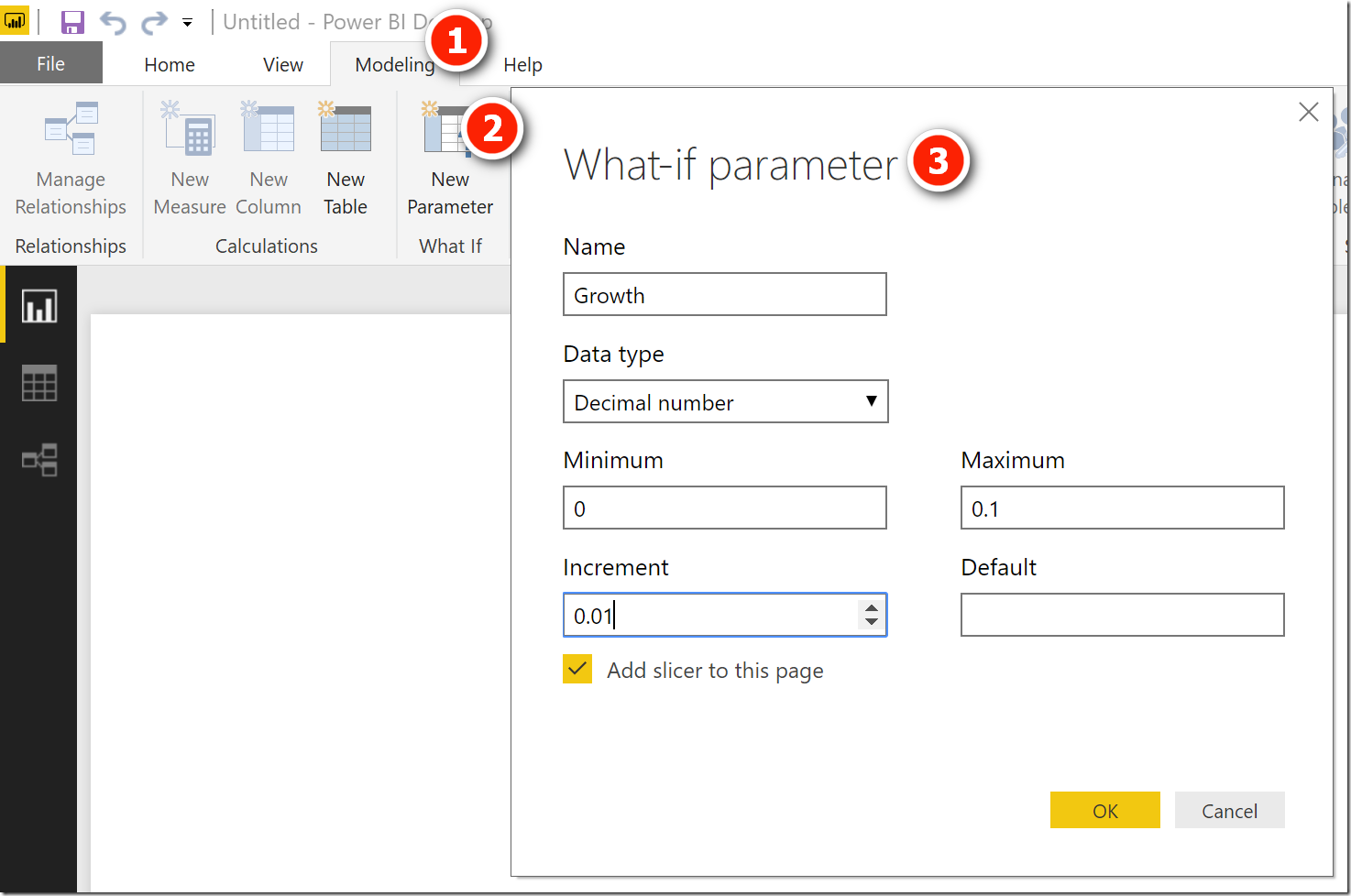After clicking OK, Power BI does a few things.

• It creates a new table (shown as 1).  This new table is generated via a DAX table function (2 below).
• The new table has a single column of values (shown as 3 below).
• The table contains a single measure that returns the currently selected value of the growth rate (4 below).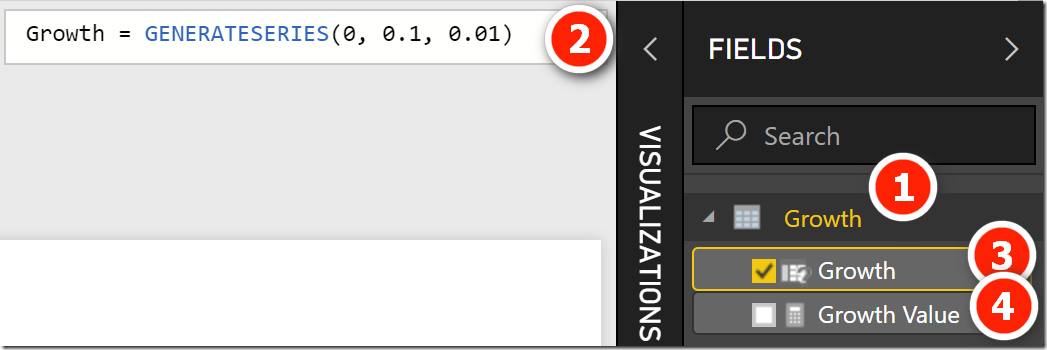Here is the measure that was auto-generated by the What If Parameter button.

`Growth Value = SELECTEDVALUE(Growth[Growth])`

And Power BI adds a slicer the the report that is connected to the table as shown below.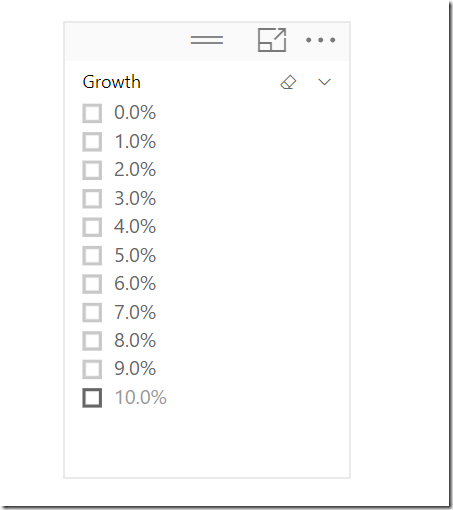Next I created a list of years, but instead of using the What If Parameter feature, I decided to simply write a new DAX table function using the GENERATESERIES table function shown above.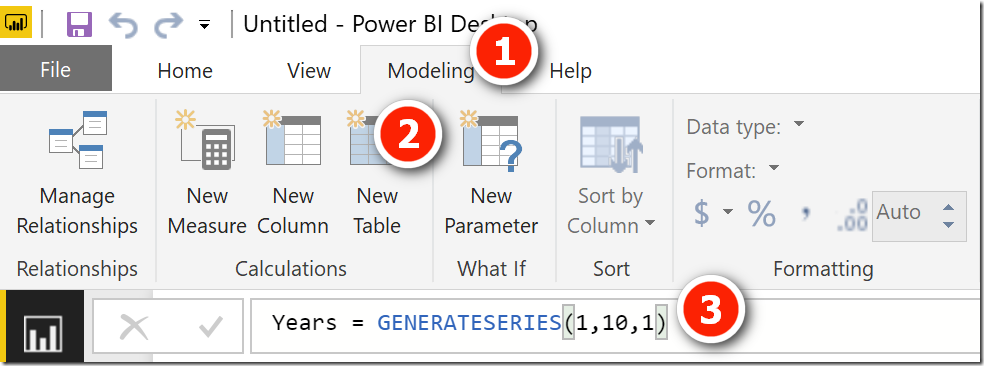I then added this new column of values into a Table onto the report canvas. I needed to change the default summarization method of this new column as I don’t want to add up the years, but instead use the years as rows on a table.  I made the change as shown below.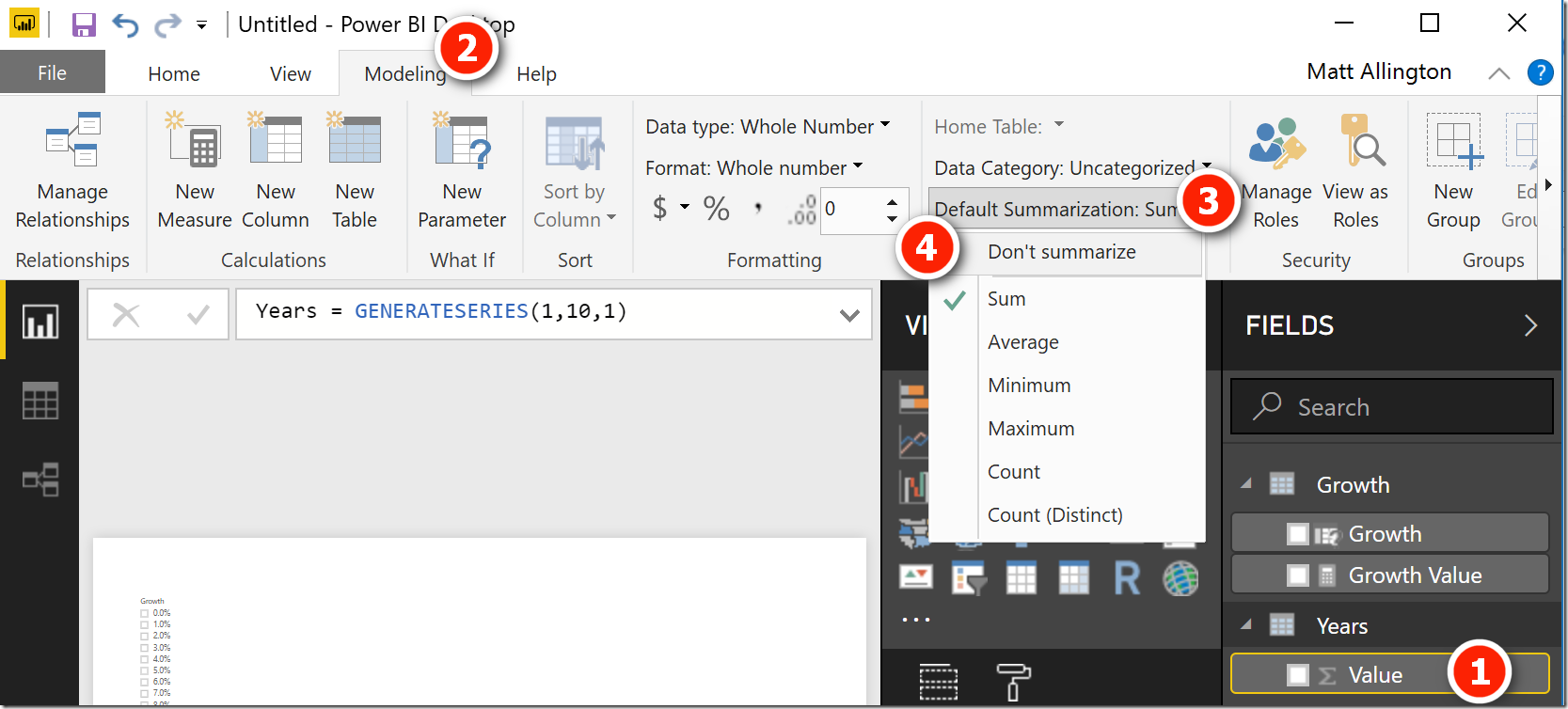I then wrote a simple “Starting Value” measure and set it to 100,000.  After the above setup, this is what I had on my report canvas.

1. A slicer connected to my disconnected rates table (created by the What If Parameters button).
2. A table connected to my Year table (table created using GENERATESERIES)
3. A card that shows the value of the Starting Value measure
4. A card that shows the current selected value in the Growth slicer.

Note, even though I have 2 tables loaded, there is no relationship between these tables – they are “disconnected tables”.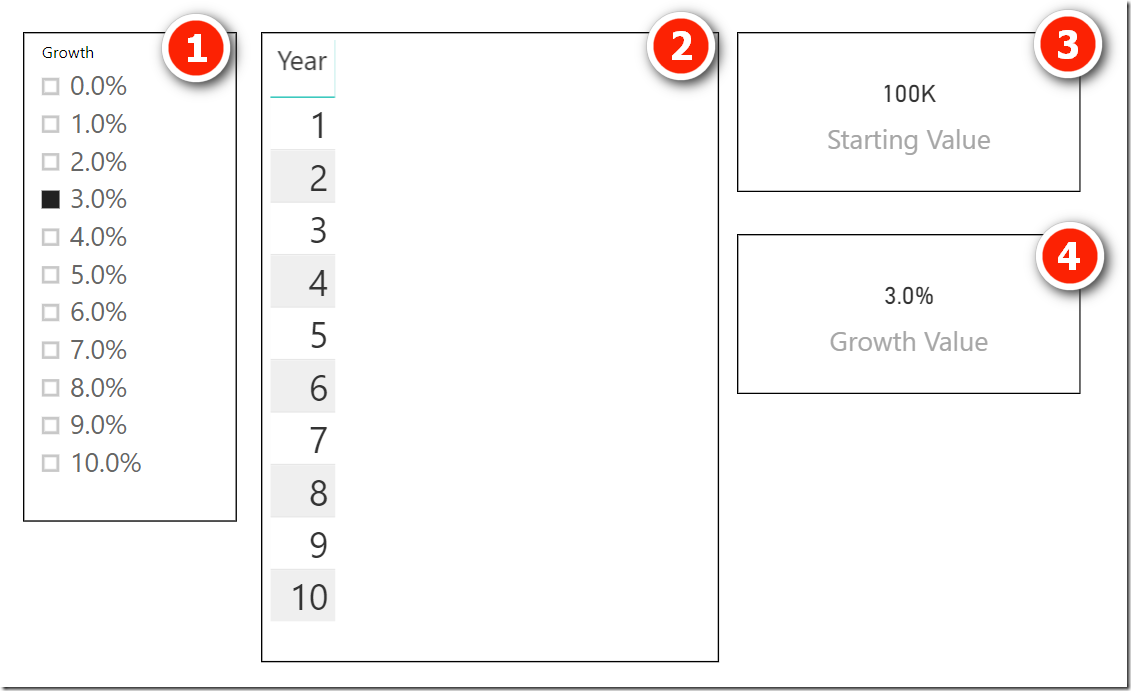## DAX formula to Forecast Future Value

The DAX formula is very similar to the one I wrote in the previous article.  Here is the formula I wrote to solve this problem in this article.

```Future Value =
VAR LatestYear =
MAX(Years[Year])
VAR UnFilteredTable =
ALL(Years)
RETURN
PRODUCTX(
FILTER(UnFilteredTable, Years[Year] <= LatestYear),
(1 + [Growth Value])
)
* [Starting Value]
```

The way this formula works is as follows.

• Lines 2 through 4 define 2 variables. I could have used SELECTEDVALUE(Years[Years]) in line 3 and I would get the same result.
• Starting from line 7, the PRODUCTX function iterates through a virtual table (line 8).  This virtual table contains all of the years up to the last selected year.  When the years are in a visual (such as a matrix or a table), the virtual table will include all years up to and including the current year in the row.  If there are no years selected, the virtual table will include all years in the the table.
• Line 9 is where the actual calculation occurs.  This line of code effectively returns (1+growth)^n where n=the number of years.
• Finally the compound growth from line 9 is multiplied by the starting value in line 11.

## Here is the result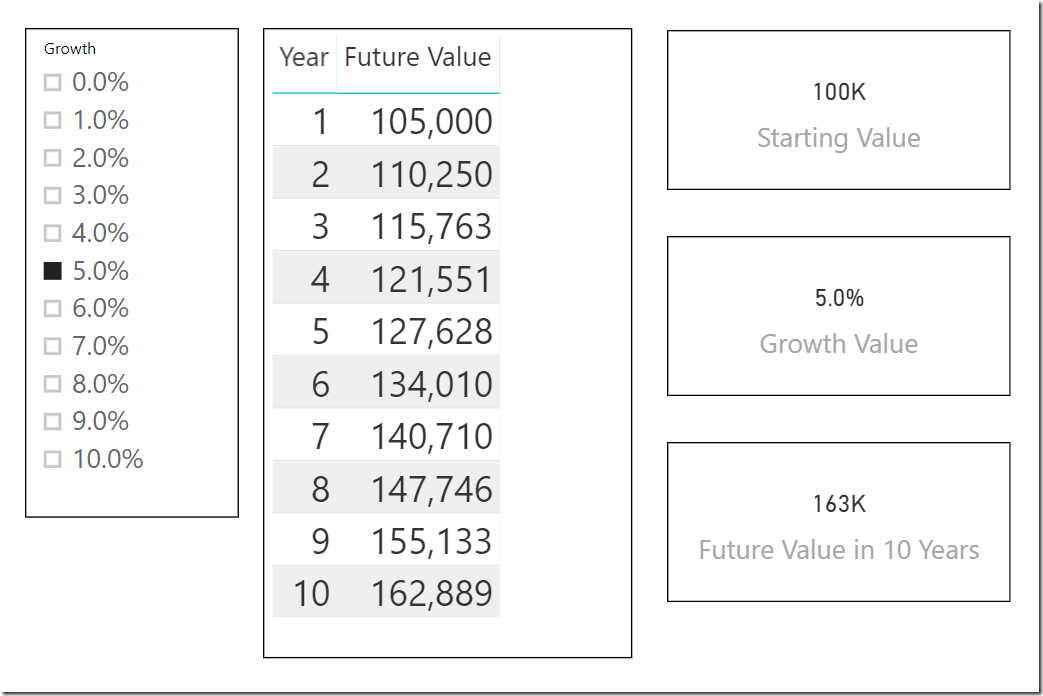Note that I renamed the bottom right card visual. This visual contains the Future Value measure (renamed to be “Future Value in 10 Years” in the visual.  Because there is no “filter” on the year, it calculates the future value over all of the data in the Years table (10 years in this case).

Here is my sample workbook if you want to take a look. future value fixed growth rate

### 8 thoughts on “User Specified Growth on an Investment using Power BI”

1.I have a table of 50 accounts & their products. These accounts buy products from distributors & sell to end customers. i have to create a dashboard which products are selling good & whatnot.

TotalSalesout = CALCULATE(SUM(PSS_Data[SalesOut]),PSS_Data[SalesType] = “Sales Out”)
TotalSTUnits = CALCULATE(SUM(PSS_Data[Units]),PSS_Data[SalesType] = “Sell Thru”)
Ratio Units = CALCULATE((DIVIDE([TotalSalesoutUnit],[TotalSTUnits])-1),PSS_Data[Report_Flag] = 1) —> Percentage (%).
output –> -500% to +500%. (+ or -20% –> Good, + or – 40% – Ok & + or – >40% is BAD)
Now I have create a slicer button for the users to with about group. When they click they should see the account, product based on ration selection

2.This is a very elegant solution, thanks for sharing!

One question I would like to add to this would be to have a future value that incorporated further investment. My example is creating a investment calculator where on top of growth through investment there is also an annual amount of money paid in – E.g an investment isa with monthly contributions.

So we need to add the contribution (From the previous 12 months to future value and then calculate growth… Is that possible, i have tried a few tweaks to what you have above but this no success.

Thanks again!

1.I can’t see why it wouldn’t work. You would need the timing of the payment of course, so this needs to be a similar table structure or the same table. Sorry, I currently don’t have time to look into this deeper.

3.I wasn’t able to understand how Future value was iteratively used to calculate the next value?

1.which part Vicki? Where did it stop making sense? I will update the post if you can let me know where it stopped making sense.

4.Thanks for this Matt. I was on page 152 of your book Supercharge Excel when I asked that question. Page 154 does explain how you, “… cannot use a measure in a ‘simple’ CALCULATE () formula” and how you need to use FILTER () for more powerful calculations (e.g., those that incorporate measures). However, I never would’ve been able to I wrap my mind around how to harness the iterative power of PRODUCTX () for problems involving both compounding and dynamic user inputs from slicers without this post.

I’ll be adapting this very valuable insight to PowerPivot for Excel so thanks also for going the extra mile and showing the use of a disconnected table (effectively a What If table) within this solution in addition to using Power BI’s What If Parameter feature as only the former is useful to me right now.

As an aside, in a world where programming languages are competing for acceptance and shelf space, I’m glad to see the increasing use of variables in DAX; perhaps those who are proficient in R and other languages should be a little less smug now as business users continue to forge ahead in a language that makes sense to us because it solves the exact kinds of problems that are most meaningful to us.

1.No worries. I agree DAX Is ground breaking for us business users, and it is only going to get better.

5.Great explanation, Matt!

Scroll to Top